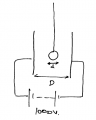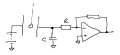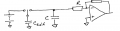# Op Amp Open Loop - Small Current Pulse - Franklin's Bell

#### HumblePhys

Joined Nov 10, 2015
3
Hi, I am a teacher working on a lab activity involving op amps and I have basic circuit knowledge i.e. stuff from college physics books. I have a parallel plate capacitor setup and I am hanging a small steel bb from a string between the plates. This is basically the same setup as Franklin's Bell. The charge from the plates jumps onto to bb whenever the pendulum swings and makes contact with one of the plates. Once it is charged it will swing back to the other plate and discharge and pickup the opposite charge and in turn swing back to the original plate and thus the process repeats.

There is around 1kV between the plates give or take a few 100 V. I am trying to use an 741 Op Amp to amplify the current once the bb touches one of the plates. At the moment I am going for maximum gain and therefore I am using it in an open loop configuration. I have not been able to detect the bb, but I have been charging an aluminum plate through friction/inductance and touching it to the plates. A spike appears on my Pasco Capstone graph that is setup to read the voltage output from the Op Amp.

However, though I get a spike, it is sometimes inconsistent and the voltage level changes after the spike. I have a kilovolt power supply with a negative/ground/positive receptacles. Right now, my positive runs directly to the left plate. Ground is connected to my right plate and in between the ground and the right plate there is a 1 kOhm resistor to create a voltage drop. My Op Amp inputs are connected to the ends of this resistor with the idea being that when there is current there will be a small voltage that can be amplified by the op amp.

My Op Amp is powered by an Extech 18V/2A power supply. There is a blue and red receptacle. I am currently using the blue as my ground but this has me confused in that I am not sure if I should tie all of my other grounds to this one. I have a ground from my Pasco voltage sensor that consists of a red and black cable. The red one is connected to the output of the Op Amp and the black one is connected to the blue output of the Extech power supply that serves as my ground. I also have the ground from by kiloV power supply but I'm not sure if this is supposed to be connected to the other two grounds. (not even sure if the blue Extech is ground)

Strange Occurrence: When I turn on the Extech to power the Op Amp, my Vout jumps to whatever voltage I have the Extech set to, even if the Op Amp inputs aren't connected. If I change the Extech voltage, the Vout changes. I've even tied the inputs together i.e. no voltage difference on the input and still the same thing. I thought that Vout =0 if there was no voltage difference.

Final thought, how likely is it that I could pick up a signal from the bb with two Op Amps if one Op Amp doesn't do the trick?

Thanks.

#### wayneh

Joined Sep 9, 2010
17,172
An op-amp requires the voltage on both inputs to be within the common mode range, which always lies between its power rails. With an old amp such as the 741 (the oldest you can find!), the inputs need to be a couple volts or so above or below the rails in order to be in range. You need to read the data sheet for the op-amp to be sure.

In your drawing, it's not clear that the ground for the input signal is at the same ground as the op-amp's power supply.

As drawn, the voltage on the inverting input will be ground, which won't work for the 741. You could try the LM358. A slightly newer amp that can sense down to the negative rail.

I think there's a good chance the voltage across that resistor might far exceed the voltage range of most op-amps. A brief spike would ruin it. Consider a zener diode to clamp the input to no more than the breakdown voltage of the zener. There may be some other good ways to protect the op-amp.

Why are you using the op-amp? I mean, what do you really want to do? The op-amp will give just a momentary spike. Maybe you'd prefer a signal output of several seconds to light an LED or buzz a buzzer?

Last edited:

#### HumblePhys

Joined Nov 10, 2015
3
What if I connected the kiloV ground to the other "grounds" i.e. the yellow line in the new picture? Also note that I had the kiloV power supply marked with a negative sign when I'm actually using the black ground receptacle (my mistake).

So what you're saying about the 741 is that I actually need a negative voltage if my input is going to be on the mV scale?

I'll probably order some of those newer amps asap.

Thanks.

#### Papabravo

Joined Feb 24, 2006
18,980
A standard operational amplifier does not amplify current. It is voltage difference in to voltage out. There is such a device as an OTA (Operation Transconductance Amplifier) that takes a voltage difference in and produces a current out. Running an opamp open loop will not be likely to produce useful results since any noise will saturate the device.

#### wayneh

Joined Sep 9, 2010
17,172
I'll probably order some of those newer amps asap.
I don't think you'd regret that, but before you do, let's talk about what the desired result is.

#### MikeML

Joined Oct 2, 2009
5,444
This is going to be tough.

I suspect that the amount of charge transferred from plate-to-ball, and ball-to-opposite plate is going to be tiny (pC) because the effective capacitance from ball to either plate will be tiny. You are effectively trying measuring the current pulse created when that tiny capacitance is charged/discharged.

The rise-time of the pulse will be fast, limited only by the contact resistance between ball and plate. The fall-time (duration) of the pulse will be a function of the time-constant of the effective series resistance in the loop between the plates and the HV power supply and the capacitance between the plates. The capacitance between the plates will also be small, likely on the order of a few pF.

To make the time constant of the recharge pulse long enough for the opamp to respond to, you will need a very fast opamp, and one that is not coming out of saturation like an open-loop comparitor...

#### HumblePhys

Joined Nov 10, 2015
3
Wayneh, I just want a voltage spike to signal when the bb contacts the plate. Im thinking I need something bigger than a bb.

Why an op amp? I thought it would be the simplest device to use to detect the pulse. A package deal. Just need a few resistors and a PS to go with it. At least I assumed so.

#### wayneh

Joined Sep 9, 2010
17,172
I agree with Mike's assessment. What if - we put a peak detector (a fast diode and a smallish capacitor) into the detector circuit. This might capture the peak and hold it long enough to be noticed by a normal op-amp. One peak detector for each polarity would be required, if you want to detect contact with both plates.

#### Tesla23

Joined May 10, 2009
516
Let's try to put some numbers on the charges and currents involved to see what's possible. This was done pretty quickly, I'm sure others will correct it if there are errors. I assume you want the ball to swing back and forth between the plates when you apply the 1000V, so you need a light ball (not a steel ball). For some numbers I used a ping pong ball (diameter 4cm, mass 2.7g), it would need to be coated in some sort of conductive paint. So for this setup:The self-capacitance of the ping pong ball is $$C_{self} = 2\pi\epsilon_0d = 2.2pF$$

so the charge on the ball is Q=CV = 2.2nC

say the plates spaced at 10cm, the electric field E = 10kV/m and the force on the charged ball is

$$F = qE = 2.2 \times 10^{-5}N$$

so if m = 2.7g, a = F/m = 0.0081m/s^2

under constant acceleration, starting from rest, the ball takes time T to get to the other side,
$$1/2 aT^2 = D$$
giving T = 5sec, so the period of the oscillation around 10sec.

The average current is Q/(2T) = 0.22nA

I would try a circuit like this:Where the charge from the ball is initially dumped into capacitor C and held for a period determined by the time constant RC. Given that you are looking at a current of the order of 220pA, one of the FET input op amps with bias currents of the order of 1pA should be fine, along with a low leakage capacitor for C.

An approximate equivalent circuit is:Where $$C_{self}$$ is the 2.2pF self capacitance of the ball. If you set C to 1nF then the 2.2nC will charge C to 2.2V, which discharges through R into the op amp. making the time constant 1ms to give the op amp plenty of time to respond, and you time to see it, gives R = 1M. If you make the op amp feedback resistor 1M you should see a 2.2V spike at the output.

•ebeowulf17

#### Tesla23

Joined May 10, 2009
516
For anyone that is interested, I just ran a quick experiment to see if my calculations were ballpark. I used a ping-pong ball roughly wrapped with alfoil on the end of a plastic rod. 'C' was a 1nF polyester capacitor across the input to my oscilloscope (so the 1Meg input resistance gives the 1ms time constant), and I used a small plate held at 64V above ground using a 30-0-30 bench supply. Running the oscilloscope on single shot, when I touched the ball on the 64V plate, then moved it over and touched it to hot end of 'C' I got a beautiful decaying exponential trace with an amplitude ranging from 70mV to 80mV. This is approximately half what my calculations predict (so not bad!). I'm not sure if there is an obvious factor of 2 in there somewhere.

Scaling this to the OP's 1000V plate suggests that he will get about a 1V pulse using my circuit, assuming the conducting sphere is around the size of a ping pong ball. A smaller sphere will give a smaller pulse and vice versa. The pulse amplitude is proportional to Cself which is proportional to the sphere radius.

•ebeowulf17 and wayneh